DATA MINING
Desktop Survival Guide
by Graham WilliamsBoosting employs a weak learning algorithm (which we identify as the learner). Suppose the dataset (data) consists of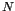entites described using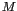variables (lines 1 and 2 of the meta-code below). Theth variable (i.e., the last variable of each observation) is assumed to be the classification of the observation. In the algorithm presented here we denote the training data (anby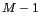matrix) as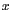(line 3) and the class associated with each observation in the training data (a vector of length) as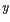(line 4). Without loss of generality we can restrict the class to be either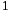(perhaps representing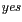) or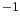(representing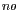). This will simplify the mathematics. Each observation in the training data is initially assigned the same weight: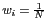(line 5).

The weak learner will need to use the weights associated with each observation. This may be handled directly by the learner (e.g., rpart takes an option to specify the Roption[]weights) or else by generating a modified dataset by sampling the original dataset based on the weights.

The first model,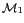, is built by applying the weak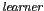to the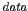with weights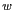(line 7)., predicting eitheror, is then used to identify the set of indicies of misclassified entities (i.e., where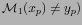, denoted as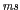(line 8). For a completely accurate model we would have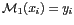. Of course the model is expected to be only slightly better than random so ms is unlikely to be empty.

A relative error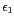foris calculated as the relative sum of the weights of the misclassified entities (line 9). This is used to calculate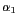(line 10), used, in turn, to adjust the weights (line 11). All weights could be either decreased or increased depending on whether the model correctly classifies the corresponding observation, as proposed by (). However, this can be simplified to only increasing the weights of the misclassified entities, as proposed by (). These entities thus become more important.

The learning algorithm is then applied to the new weightedwith theexpected to give more focus on the difficult entities whilst building this next model,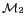. The weights are then modified again using the errors from. The model building and weight modification is then repeated until the new model performs no better than random (i.e., the error is 50% or more: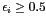), or is perfect (i.e., the error rate is 0% andis empty), or perhaps after a fixed number of iterations.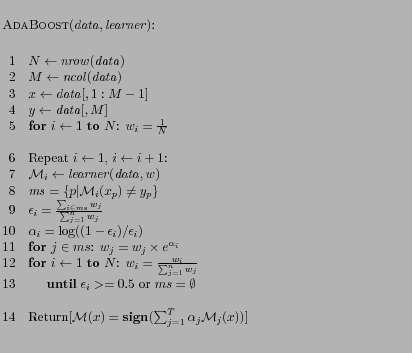The final model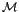(line 14) combines the other models using a weighted sum of the outputs of these other models. The weights,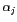, reflect the accuracy of each of the constituent models.

A simple example can illustrate the process. Suppose the number of training entities,, is 10. Each weight,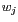, is thus initially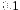(line 5). Imagine the first model,, correctly classifies the first 6 of the 10 entities (e.g.,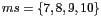), so that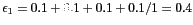. Then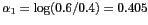, and is the weight that will be used to multiply the results from this model to be added into the overall model score. The weights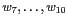then become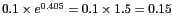. That is, they now have more importance for the next model build. Suppose now thatcorrectly classifies 8 of the entities (with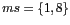), so that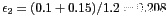and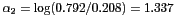. Thus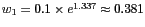and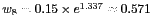. Note how record 8 is proving particularly troublesome and so its weight is now the highest.

We can understand the behaviour of the function used to weight the models (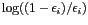) and to adjust the observation weights (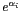) by plotting both of these for the range of errors we expect (from 0 to 0.5 or 50%).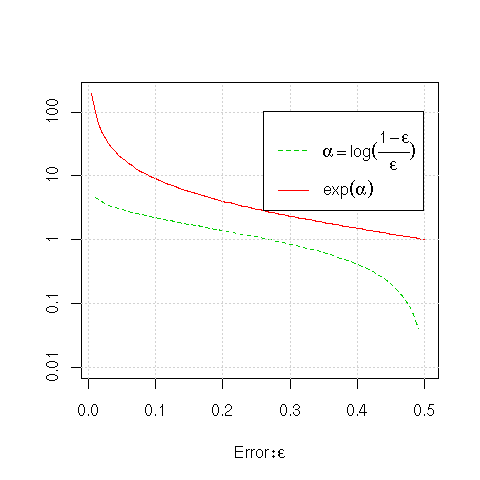plot(function(x) exp(log((1-x)/x)), xlim=c(0.01, 0.5), ylim=c(0.01, 200), xlab=expression(Error: epsilon), ylab="", log="y", lty=1, col=2, yaxt="n") plot(function(x) log((1-x)/x), add=TRUE, lty=2, col=3) axis(side=2, at=c(0.01, 0.1, 1, 10, 100), c(0.01, 0.1, 1, 10, 100)) grid() exp.leg <- expression(alpha == log(over(1-epsilon, epsilon)), exp(alpha)) legend("topright", exp.leg, lty=2:1, col=3:2, inset=c(0.04, 0.1)) 

First, looking at the value of the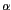's (), we see that for errors close to zero, that is, for very accurate models, the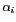(i.e., the weight that is used to multiply the results from the model) is very high. For an error of about 5% the multiplier is almost 3, and for a 1% error the multiplier is about 4.6. With an error of approximately 27% (i.e.,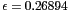) the multiplier is close to 1. For errors greater than this the model gets weights less than 1, heading down to a weight of 0 at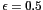.
In terms of building the models, the observation weights are multiplied by. If the model we have just built (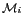) is quite accurate (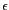close to 0) then fewer entities are being misclassified, and their weights are increased significantly (e.g., for a 5% error the weight is multiplied by 19). For inaccurate models (asapproaches 0.5) the multiplier for the weights approaches 1. Note that if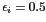the multiplier is 1, and thus no change is made to the weights of the entities. Of course, building a model on the same dataset with the same weights will build the same model, thus the criteria for continuing to build a model tests that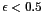.